Orient

Moves or copies, rotates, and scales objects using two reference and two target points.

Steps:

1. Select

the objects.

2. Pick

two reference points.

3. Markers are drawn at the reference points.

4. Pick two target points.

5. The first and second reference points will match these locations.

The objects are moved, scaled and rotated so the reference points transform to the target points.

Options

Copy

Scale

No

The second target point defines the direction of the transformation, but objects remain the same size.

1D

Scale the object only along the axis between the target points.

3D

The second target point defines the direction of the transformation and the scale factor for the objects.

### History enabled…

Changing the input objects changes the output objects.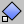Transform > Orient: 2 PointsTransform > Orient > 2 Points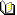Related topics…

Orient3Pt

Moves or copies and rotates objects using three reference and three target points.

Steps:

1. Select

the objects.

2. Pick

the first reference point.

3. Pick the second reference point.

4. This defines a base direction from the first reference point.

5. Pick the third reference point.

6. Pick the first target point.

7. This corresponds to the first reference point.

8. Pick the second target point.

9. This corresponds to the second reference point.

10. Pick the third target point.

11. This corresponds to the third reference point.

Option

Copy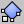Transform > Orient: 3 Points (Right click)Transform > Orient > 3 PointsRelated topics…

OrientCrvToEdge

Copies and aligns a curve to a surface edge.

Steps:

1. Select

a curve near an end.

2. Select a target surface edge.

3. Pick

target edge points along the edge to align the curve.

Notes

• If the curve does not start on the edge, it is rotated so the picked end of the curve is tangent to the surface and perpendicular to the edge.

• If the curve already starts on the edge, it is copied to a new location on the edge with the same orientation as the original curve had to the surface.

• If the curve tangent at the picked location is in the same direction as the active view’s construction plane, the curve is not re-oriented to be tangent with the surface.

Options

Copy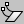Transform > Orient Curve to EdgeTransform > Orient > Curve to EdgeRelated topics…

OrientOnCrv

Moves or copies and rotates objects along a curve using the curve direction for orientation.

Steps:

1. Select

objects.

2. Pick

a base point.

3. Select the orientation curve.

4. Pick a new base point on the curve.

Options

Copy

Perpendicular

Orients the object perpendicular to the curve. The perpendicular plane is determined by the current construction plane when the base point is picked.

XFlip/YFlip

Reverses the orientation relative to the original orientation to the construction plane

Rotate

Pick an angle or two reference points to rotate the curve.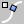Transform > Orient > On CurveTransform > Orient > On CurveRelated topics…

OrientOnSrf

Steps:

1. Select

the objects.

2. Pick

two reference points.

3. The first reference point defines the new origin of the reference plane.

The vector created by second and first reference points defines the reference plane’s x-axis.

In addition, the distance between the first and second reference points is used as input when scaling the objects.

The z-direction of the current construction plane determines the “up” direction for the object. The up direction will then follow the surface normal direction on the target surface.

4. Select the surface.

5. Pick target points on the surface.

6. As you move the cursor over the surface, you will see a dynamic preview image of the transformed objects being reoriented by the varying normal direction of the surface.

Options

OnSurface

Sets the initial base point on the surface. This option is useful if the object is already on the target surface but must be copied or moved.

The option uses initial base points on the target surface and uses the surface normal rather construction plane normal to determine the initial orientation of the object.

Flip

Scale

Prompt

Prompts to pick

a scale a for each copy.

Uniform

Sets the same scale for the x-, y-, and z-directions.

X/Y/Z

Sets a separate scale for each direction.

Rotation

Prompt

Prompts to pick

a rotation angle for each copy.

Angle

Specify an angle of rotation. Rotation is around the surface normal at the target point.

Copy objects

Rigid

Yes

Orients a set of objects without deforming the individual objects.

No

Objects deform as they are oriented.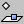Transform > Orient on SurfaceTransform > Orient > On SurfaceRelated topics…

Splop

Copies, rotates, scales, and wraps objects on a surface, like pottery sprigging or appliqué.

Steps

1. Select objects.

2. Draw a sphere around the input object(s).

3. This is the reference sphere that determines the size and orientation.

4. Select a surface to wrap the objects onto.

5. Pick a point on the selected surface.

6. Drag out a sphere.

Options

Copy

Rigid

Flip

Use if your object is upside down.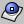UDT > SplopNoneRelated topics…